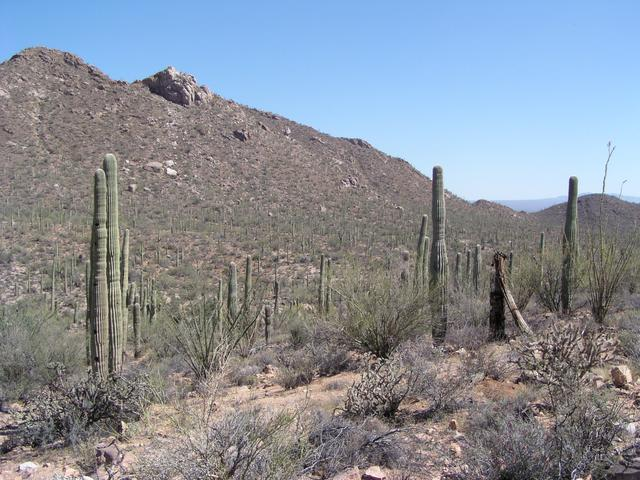Fall 2018, Siena College

Lecture 28: Trees
Date: Friday, November 16, 2018

Agenda

• Announcements
• Quiz in class Monday
• there will be a variation of this question: list reasons why it is good to implement and use more restrictive structures
• Introduction to tree structures
• concept and terminology
• a first binary tree implementation
• A general-purpose binary tree
• Tree traversals

Tree Examples

Most trees have branches (except in Tucson):Around here, our trees branch:Terminology

• expression tree
• empty tree
• tree node
• root node
• subtree
• edge
• children
• leaves/leaf nodes
• interior nodes
• parent
• forest of trees
• simple path
• path length
• height of a node
• height of the tree
• depth of a node
• degree of a node
• level of a node
• binary tree
• left/right subtrees
• full tree
• complete tree
• perfect tree

Examples